Brain Builder - Math Edition 3.0 Over 500 million math puzzles in a multimedia game. Has 16 skill levels. Shareware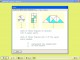Fraction Shape-Up 1.00 Interactive software that helps students understand and work with fractions. Shareware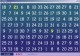Math Worm 2.0 A math puzzle where your worm navigates a maze completing arithmetic equations. SharewareMultiplication 1.0.4c Multiplication is a simple math multiplication skill for Mac OS X. FreewareMath-Flight 2 2.3 Fly High while having fun learning basic mathematics with Math Flight! Shareware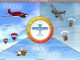Math Flight 2.3 Fly High while having fun learning basic mathematics with Math Flight! Shareware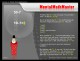Mental Math Master 1.2 Mental math skills in 3 minutes a day with this multimedia tutor. Demo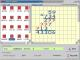Math 1 Educational math software for school or personal. SharewareMath Squeeze 1.0.1 Exciting Math game for kids. Great for beginners to experts. SharewareMath Basics 0.1 Math Basics is a simple math quiz application. Freeware